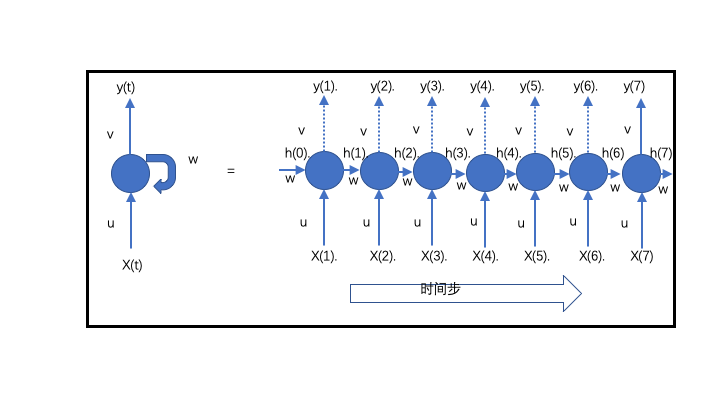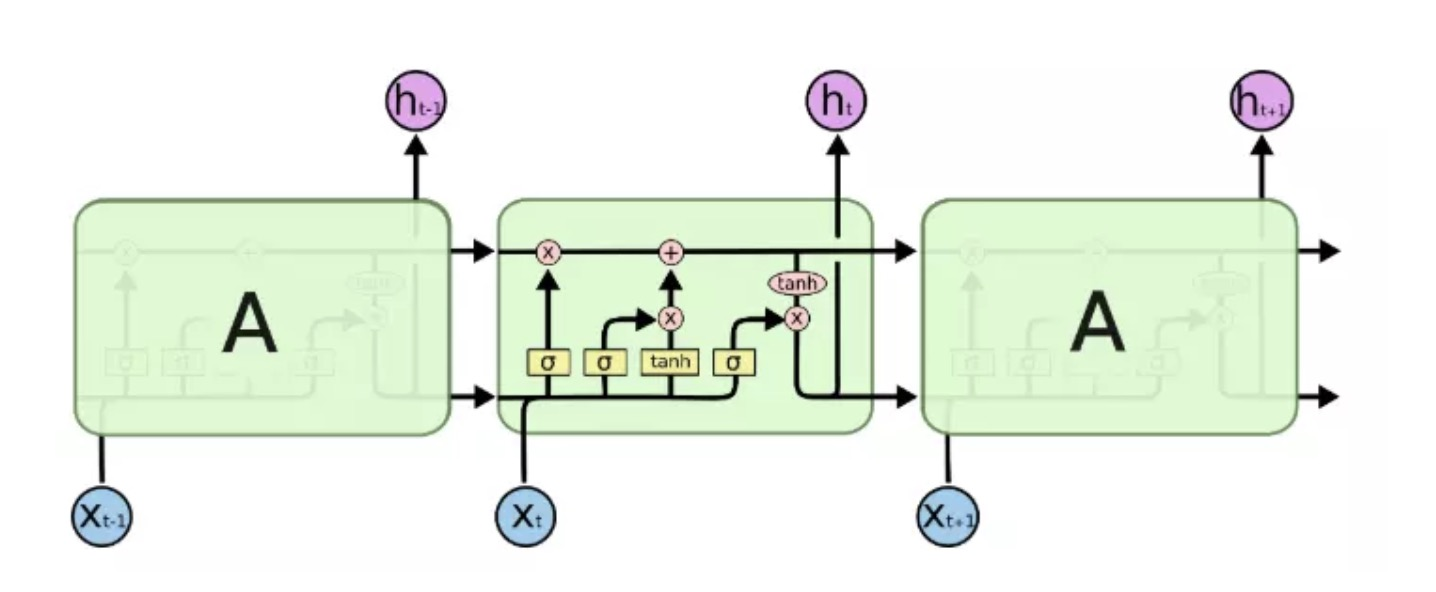# 从RNN到LSTM小记

### 1.SimpleRNN

RNN用隐藏状态或记忆引入后序数据对前面数据的依赖，以保存当前关键信息。RNN可图形化为左图的结构，其中

• X(t)为时间步t的输入数据，即每一天的天气数据特征（特征具体是什么暂时不考虑）
• y(t)为对应时间步的输出。同时，输出的一部分（隐藏状态h(t)）反馈给神经单元，以在接下来的时间步t+1使用。即隐藏状态使序列信息得到保留以作用于下一个输入。
• u,v,w为权重矩阵。

$$h(t) = tanh(W*h(t-1) + U*x(t))$$

$$y(t) = relu(V*h(t))$$

### 2.LSTMLSTM 按3个时间步展开如上图所示. LSTM单元内部结构比RNN更加复杂。LSTM单元内部结构如下图：• i–输入门： $$i = sigmoid(Wi*[h(t-1),x(t)] + bi)$$
• f–遗忘门： $$f = sigmoid(Wf*[h(t-1, x(t)] + bf)$$
• o–输出门： $$o = sigmoid(Wo*[h(t-1, x(t)] + bo)$$
• $$g = tanh(Wg*[h(t-1),x(t)] + bg)$$
• $$c(t) = c(t-1).* f + g.* i$$
• $$h(t) = tanh(c(t)) .* o$$

i,f,o三者通过sigmoid激活函数转换成0到1之间的数值，来作为一种门控状态.sigmoid函数调节这些门使其输出0和1之间的值，将产生的输出向量与另一个向量进行元素乘，以决定第二个向量有多少部分可以通过. g则是将结果通过tanh激活函数将转换成-1到1之间的值,g输入数据，而不是门控信号。 LSTM中具有两个状态,c(t)为长期记忆单元：变化慢，由c(t-1)加上一些数值，h(t)为短期记忆单元：不同节点下往往会有很大的区别。

1.忘记阶段： 遗忘门定义了h(t-1)传进来的输入有多少可以通过。简单来说就是会 “忘记不重要的，记住重要的”。遗忘门设为0时表示忽略所有就记忆。

2.选择记忆阶段： 输入门定义为当前输入x(t)新计算出来的状态有多少可以通过。

3.输出阶段：这个阶段输出门将决定哪些将会被当成当前状态的输出。

### 3.LSTM参数计算

# 本例中，假设units 128, features 1, 公式可得一层LSTM参数为 66560

n_timesteps = 7
n_features = 1
model = Sequential()
model.add(LSTM(128, activation='relu', input_shape=(n_timesteps, n_features)))
model.add(Dense(1))
model.add(Activation("relu"))
model.summary()### 4.keras LSTM 输入

• samples为样本个数，即为Batch_size
• timesteps为时间步数，即控制一个LSTM单元在一个样本上会执行多少次。对于序列数据而言，即依赖于前多少个数据。比如我们用7天预测之后一天，时间步即为7.用5天即为5.
• features为每一个时间步的特征的纬度，此处的纬度为一维向量(所以在将LSTM和CNN结合时都需要将CNN输出flatten). 本例中假如只根据前7天的温度来预测之后一天的温度.那么每一天的特征只有温度，则features为1. 引入更多特征，features即为特征向量的长度。

# 假如每个时间步，特征纬度为1，一个样本数据如下
data = np.array([1, 2, 3, 4, 5, 6, 7])
data = np.reshape(data, (1, 7, 1))

# 特征纬度为3,一个样本的数据格式如：
# shape(7, 3)
data = [[1, 2, 3],
[2, 4, 6],
[4, 3, 5],
[7, 5, 9],
[1, 2, 3],
[6, 3, 9],
[3, 5, 7]]
# 若一次要训练多个样本，如samples=4,则读取的data shape为（28，3）
data = np.array(...)
data = np.reshape(data, (4, 7, 3))